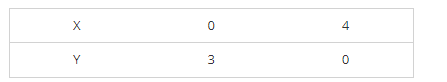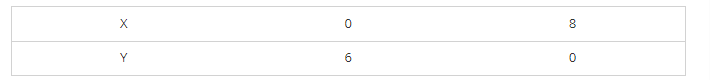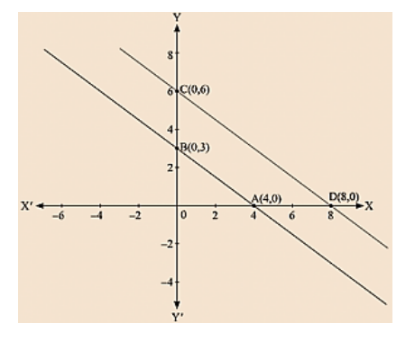# The path of a train A is given by the equation 3x + 4y -12 = 0

Question:

The path of a train A is given by the equation 3x + 4y -12 = 0 and the path of another train B is given by the equation 6x + 8y - 48 = 0. Represent this situation graphically.

Solution:

We are given the path of train A, 3x + 4y - 12 = 0

We get,

$y=\frac{12-3 x}{4}$

Now, substituting x = 0 in

$y=\frac{12-3 x}{4}$

we get y = 3

Substituting x = 4 in

$y=\frac{12-3 x}{4}$

we get y = 0

Thus, we have the following table exhibiting the abscissa and ordinates of points on the line represented by the given equationPlotting A(4, 0) and E(0, 3) on the graph and by joining the points,

we obtain the graph of equation 3x + 4y - 12 = 0.

We are given the path of train B, 6x + 8y - 48 = 0

We get,

$y=\frac{48-6 x}{8}$

Now, substituting x = 0 in

$y=\frac{48-6 x}{8}$

we get y = 6

Substituting x = 8 in

$y=\frac{48-6 x}{8}$

we get y = 0

Thus, we have the following table exhibiting the abscissa and ordinates of points on the line represented by the given equationPlotting C (0, 6) and D (8, 0) on the graph and by joining the points. We obtain the graph of equation 6x + 8y – 48 = 0